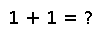# scale!

``````..  scale!(A, b)
scale!(b, A)

Scale an array ``A`` by a scalar ``b``, similar to :func:`scale` but
overwriting ``A`` in-place.

If ``A`` is a matrix and ``b`` is a vector, then ``scale!(A,b)``
scales each column ``i`` of ``A`` by ``b[i]`` (similar to
``A*diagm(b)``), while ``scale!(b,A)`` scales each row ``i`` of
``A`` by ``b[i]`` (similar to ``diagm(b)*A``), again operating in-place
on ``A``.``````

## Examples

Checking you are not a robot: